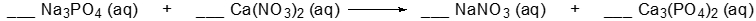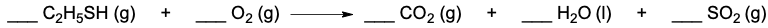Start typing, then use the up and down aroows to select an option from the list.

# GOB Chemistry

Learn the toughest concepts covered in your GOB - General, Organic, and Biological Chemistry class with step-by-step video tutorials and practice problems.

Next ChapterClassification & Balancing of Chemical Reactions

# Balancing Chemical Equations (Simplified)

Next TopicBalancing Chemical Equations require the number of atoms to be the same on both sides of the arrow.

## Balancing Chemical Equations

1

### concept

Balancing Chemical Equations (Simplified) Concept 11m
Play a video:
0
2

### example

Balancing Chemical Equations (Simplified) Example 14m
Play a video:
0
3
Problem

Write the balanced equation for the following by inserting the correct coefficients in the blanks.4
Problem

Determine the total sum of the coefficients after balancing the following equation.